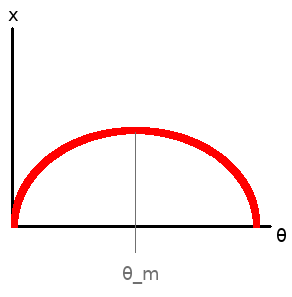# Background

I recently discovered how to write equations in my Hugo website using Mathjax (credit goes to kunlei for the instructions!). In order to demonstrate the awesomeness of this tool (and also to refresh my memory on basic Physics concepts I have not used in a long time), let me demonstrate why 45 degrees is the ideal angle to throw something at maximum range.

# Solution

First off, for simplification purposes, let us suppose the friction due to air resistance is negligible.

If we use a referential where the $x$ axis is parallel to the ground and $y$ axis is perpendicular to the ground, what we want to maximize is the function $x(\theta)$, i.e. the distance travelled by our object as a function of the angle at which we throw it. Let’s define $\theta$ as the angle we throw the object with respect to the $y$ axis.

Intuitively, we know that an object thrown with $\theta=0$ wouldn’t travel any distance because it would reach the ground immediately. Similarly, we know that throwing an object with $\theta=90$ would have the same result: the object would go up in the air, and in the absence of wind, would fall back exactly from where it left the ground initially. Thus, the angle that maximizes the distance ($\theta_m$) is an angle between 0 and 90.

Naively, we can assume the $x(\theta)$ function looks a little something like this:The travelled distance $x$ as a function of the angle $\theta$

Because the thrown object moves in a fluid motion that follows the laws of kinematics, it is reasonable to expect that $\theta_m$ is found exactly where the derivative of $x(\theta)$ becomes 0, that is to say $\frac{dx}{d\theta}=0$.

So, our game plan is to find the equation of $x(\theta)$ and find which value of $\theta$ makes $\frac{dx}{d\theta}=0$.

A well known equation of kinematics is $d=v_i\Delta t + \frac{1}{2}a\Delta t^2$. When applied to the movement of our thrown object over the $x$ axis, this becomes

$$x=v_{ix}\Delta t + \frac{1}{2} a_x \Delta t^2$$ $$\Leftrightarrow x= v_{ix}\Delta t \quad \text{because a_x = 0}$$

By expressing the velocity in terms of $x$ and $y$ components (see figure above), we can re-express $x$ as

$$x = v\cos\theta\Delta t$$

Ah ha! We are starting to unfold how $x$ is dependent of $\theta$ explicitly. However, inside $\Delta t$, there is a hidden dependency on $\theta$. Indeed, you can imagine, if we throw an object at $\theta=0$, its airborne time will be null. On the other hand, if we throw that same object at $\theta=90$, its airborne time will certainly be $>0$ (assuming $v \neq 0$). Thus, what is the expression for $\Delta t (\theta)$?

Let’s use the kinematics equation in the $y$ axis to get some insight.

$$\Delta y = v_{iy}\Delta t+\frac{1}{2}a_y\Delta t^2$$

Because we throw the object from a flat plane and we suppose it falls at the same height from which it was launched (i.e. $\Delta y = 0$), we get

$$0 = v_{iy}\Delta t + \frac{1}{2}a_y\Delta t^2$$ $$0 = v\sin\theta\Delta t+\frac{a_y}{2}\Delta t^2$$ $$0 = \Delta t(v\sin\theta + \frac{a_y}{2}\Delta t) \quad \text{(here we divide by \Delta t \neq 0)}$$ $$0 = v\sin\theta + \frac{a_y}{2}\Delta t$$ $$\Leftrightarrow \Delta t = \frac{-2v\sin\theta}{a_y}$$

Hurray, we found our equation for $\Delta t (\theta)$, it is $\Delta t(\theta) = \frac{-2v\sin\theta}{a_y}$

We can thus substitute this value in the earlier equation we found for $x(\theta)$:

$$x(\theta)=v\cos\theta\Delta t(\theta) = v\cos\theta\left(\frac{-2v\sin\theta}{a_y}\right) = \frac{-2v^2\cos\theta\sin\theta}{a_y}$$

Let us derivate this equation:

$$\frac{dx}{d\theta} = \frac{-2v^2}{a_y}\left(-\sin^2\theta+\cos^2\theta\right) = \frac{2v^2}{a_y}\left(\sin^2\theta-\cos^2\theta\right)$$

We are interested in finding $\theta_m$ for which $\frac{dx}{d\theta}=0$. In other words,

$$\frac{dx}{d\theta} = 0 = \frac{2v^2}{a_y}\left(\sin^2\theta_m-\cos^2\theta_m\right)$$ $$\Leftrightarrow \sin^2\theta_m = \cos^2\theta_m$$ $$\Leftrightarrow \frac{\sin^2\theta_m}{\cos^2\theta_m} = 1$$ $$\Leftrightarrow \frac{\sin\theta_m}{\cos\theta_m} = \tan\theta_m = 1$$ $$\Leftrightarrow \theta_m = \arctan(1) = \frac{\pi}{4} = 45^o \quad\blacksquare$$

And there we have it, proof that the angle that maximizes the distance travelled by a thrown object is $\theta_m = 45^o$.

# Conclusion

This was a fun trip down memory lane for me. I might do this again sometime! As you can see, Mathjax is very good at rendering equations in HTML. Let me know if you found this interesting (maybe you’re a LaTeX user, or maybe you’re learning physics/maths). 😃